#### 期刊菜单

High Precision 3D Scene Reconstruction Based on Monocular Vision
DOI: 10.12677/AIRR.2018.73013, PDF, HTML, XML, 下载: 1,067  浏览: 3,518  科研立项经费支持

Abstract: In recent years, along with the rapid updating of computer hardware, the processing capability of computer is also increasing. At the same time, 3d scene reconstruction technique has become more and more mature and we can get 3d model data for scenarios more easily than ever before. Now, in the 3d reconstruction technology based on monocular and binocular, monocular technology is simpler to operate than binocular technology and more convenient to acquire materials and more favorable to the market. This paper focuses on monocular based 3D reconstruction, the algorithm is used to reconstruct the 3d scene with a fast NCC algorithm based on the cumulative diagram. This paper improves the classic NCC similarity measures to reduce the computation time. Seed pixel expansion algorithm is presented to choose the initial seed pixels, use parallax to make window comparisons to obtain high confidence seed pixels, therefore, the mismatches of the parallax figure are greatly reduced. Experiments show that the method can reconstruct precise and clear 3d scenarios.

1. 引言

2. 相关工作

3. 场景高精度三维模型重建算法

3.1. 算法概述

3.2. 摄像机内参数标定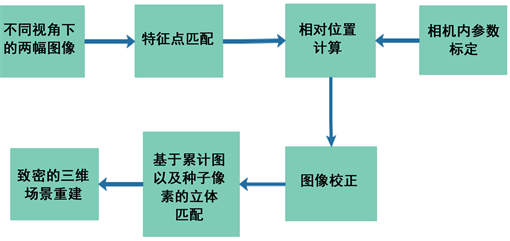Figure 1. Algorithm flow diagram

3.3. 图像采集和特征匹配

3.4. 图像间相对位置计算

3.5. 图像校正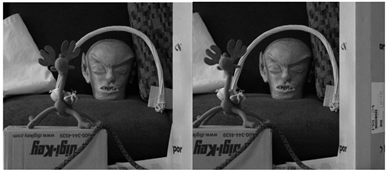Figure 2. The diagrams used in the experiment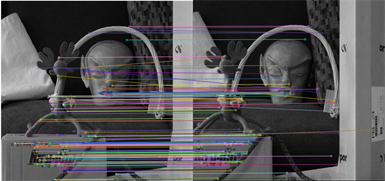Figure 3. The result of SIFT feature matching of two corrected images

3.6. 立体匹配

3.6.1. 基于累积图的快速NCC匹配代价计算

NCC匹配代价为：

$C\left(p,d\right)=\frac{\underset{\left(x,y\right)\in {W}_{p}}{\sum }\left({I}_{1}\left(x,y\right)-{\stackrel{¯}{I}}_{1}\left({p}_{x},{p}_{y}\right)\right)\cdot \left({I}_{2}\left(x+d,y\right)-{\stackrel{¯}{I}}_{2}\left({p}_{x}+d,{p}_{y}\right)\right)}{\sqrt{\underset{\left(x,y\right)\in {W}_{p}}{\sum }{\left({I}_{1}\left(x,y\right)-{\stackrel{¯}{I}}_{1}\left({p}_{x},{p}_{y}\right)\right)}^{2}\cdot \underset{\left(x,y\right)\in {W}_{p}}{\sum }{\left({I}_{2}\left(x+d,y\right)-{\stackrel{¯}{I}}_{2}\left({p}_{x}+d,{p}_{y}\right)\right)}^{2}}}$ (1)

$i{i}_{k}\left({W}_{p}\right)=\underset{\left(x,y\right)\in {W}_{p}}{\sum }{I}_{k}\left(x,y\right)$ (2)

${I}_{22}\left(x,y\right)={I}_{2}\left(x,y\right)×{I}_{2}\left(x,y\right)$ (3)

${D}_{r}={D}_{\mathrm{max}}-{D}_{\mathrm{min}}+1$ (4)

${I}_{12}\left(x,y\right)={I}_{1}\left(x,y\right)×{I}_{2}\left(x+d,y\right)$ (5)

$C\left(p,d\right)=\frac{i{i}_{12}\left({W}_{p}\right)-\frac{1}{|{W}_{p}|}\cdot i{i}_{1}\left({W}_{p}\right)\cdot i{i}_{2}\left({W}_{p+d}\right)}{\sqrt{\left[i{i}_{11}\left({W}_{p}\right)-\frac{1}{|{W}_{p}|}\cdot i{i}_{1}{\left({W}_{p}\right)}^{2}\right]\cdot \left[i{i}_{22}\left({W}_{p+d}\right)-\frac{1}{|{W}_{p}|}\cdot i{i}_{2}{\left({W}_{p+d}\right)}^{2}\right]}}$ (6)

$O\left(WH\right)+O\left(WH\right)+\left(O\left(WH\right)+O\left(WH\right)\right)+\left(O\left(WH\right)+O\left(WH\right)\right)=O\left(WH\right)$ (7)

3.6.2. 种子像素提取算法

$\left\{\begin{array}{l}k×C\left({p}_{x},{p}_{y},disp\right)\ge C\left({p}_{x},{p}_{y},d\right),\forall d\ne disp\\ k×C\left({p}_{x},{p}_{y},disp\right)\ge C\left({{p}^{\prime }}_{x},{p}_{y},{d}^{\prime }\right),{{p}^{\prime }}_{x}+{d}^{\prime }={p}_{x}+disp,{d}^{\prime }\ne disp\end{array}$ (8)

${S}_{u}=\left\{\left({p}_{x},{p}_{y},disp\right)|{p}_{y}<\frac{H}{2}\right\}$ (9)

${S}_{d}=\left\{\left({p}_{x},{p}_{y},disp\right)|{p}_{y}\ge \frac{H}{2}\right\}$ (10)

$\underset{i={p}_{x}-w}{\overset{{p}_{x}+w}{\sum }}\underset{j={p}_{y}-w}{\overset{{p}_{y}+w}{\sum }}{\left({D}_{u}\left(i,j\right)-{D}_{d}\left(i,j\right)\right)}^{2}=0$ (11)

$P\left({p}_{x},{p}_{y},d\right)\propto C\left({p}_{x},{p}_{y},d\right)$ (12)

$\begin{array}{l}{d}^{\prime }={\mathrm{arg}}_{disp}\mathrm{max}\left(C\left({{p}^{\prime }}_{x},{{p}^{\prime }}_{y},disp\right)\right)\\ disp\in \left\{d-1,d,d+1\right\}\end{array}$ (13)

$\begin{array}{l}{d}^{\prime }={\mathrm{arg}}_{disp}\mathrm{max}\left(C\left({{p}^{\prime }}_{x},{{p}^{\prime }}_{y},disp\right)\right)\\ disp\in \left\{d-1,d,d+1,{d}_{1}\right\}\end{array}$ (14)

4. 实验结果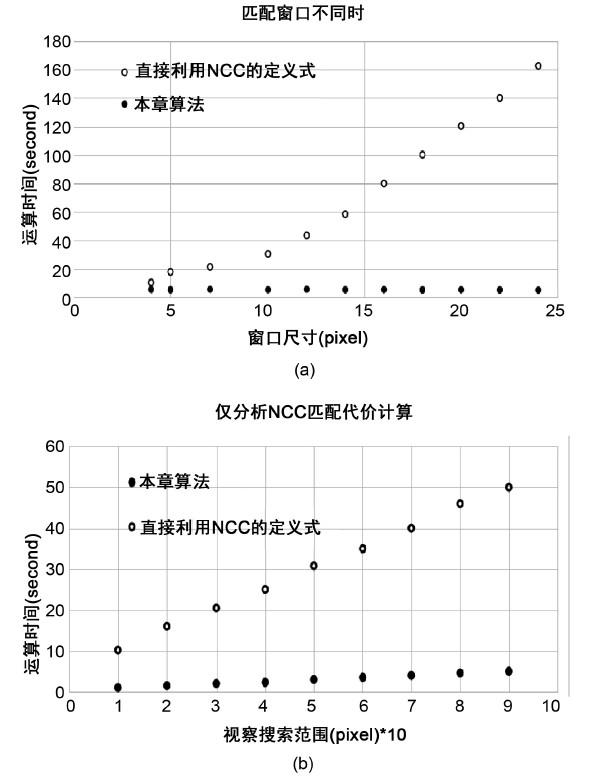Figure 4. Comparison of computation time of NCC matching cost method. (a) Match the operation time different windows sizes. (b) Comparison of only NCC matching cost calculation is analyzed

5. 重建结果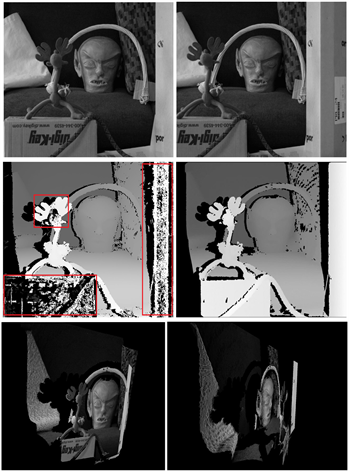Figure 5. Reconstruction results of NO. 1 indoor scene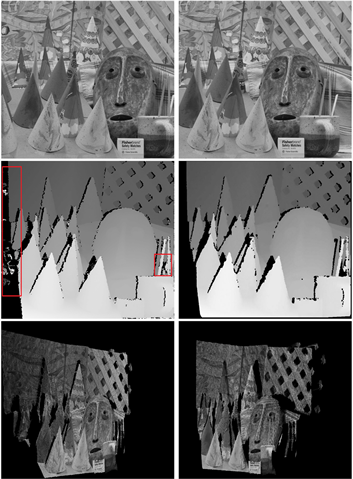Figure 6. Reconstruction results of NO. 2 indoor scene

6. 结论

NOTES

*通讯作者。

  Cyberware (2008) Cyberware Web Page. http://www.cyberware.com  Konica (2008) Konica Minolta 3-D Digitizer Web Page. http://www.minolta3d.com  Scharstein, D. and Szeliski, R. (2003) High-Accuracy Stereo Depth Maps Using Structured Light. 2003 IEEE Computer Society Conference on Computer Vision and Pattern Recognition, Madison, WI, 18-20 June 2003, 195-202. https://doi.org/10.1109/CVPR.2003.1211354  Neugebauer, P. and Klein, K. (1999) Texturing 3D Models of Real World Objects from Multiple Unregistered Photographic Views. Eurographics, 18, 245-256.  Scharstein, D. and Szeliski, R. (2002) A Taxonomy and Evaluation of Dense Two-Frame Stereo Correspondence Algorithms. International Journal of Computer Vision, 47, 7-42. https://doi.org/10.1023/A:1014573219977  Ogale, A. and Aloimonos, Y. (2007) A Roadmap to the Integration of Early Visual Modules. International Journal of Computer Vision, 72, 9-25. https://doi.org/10.1007/s11263-006-8890-9  Pollefeys, M., Van Gool, L. and Vergauwen, M. (2004) Visual Modeling with a Hand-Held Camera. International Journal of Computer Vision, 59, 207-232. https://doi.org/10.1023/B:VISI.0000025798.50602.3a  Snavely, N., Seitz, S.M. and Szeliski, R. (2008) Modeling the World from Internet Photo Collections. International Journal of Computer Vision, 80, 189-210. https://doi.org/10.1007/s11263-007-0107-3  Geiger, A., Ziegler, J. and Stiller, C. (2011) StereoScan: Dense 3d Reconstruc-tion in Real-Time. Intelligent Vehicles Symposium, Baden-Baden, 5-9 June 2011, 963-968. https://doi.org/10.1109/IVS.2011.5940405  Shen, S.H. (2013) Accurate Multiple View 3D Reconstruction Using Patch-Based Stereo for Large-Scale Scenes. IEEE Transactions on Image Processing, 22, 1901-1914. https://doi.org/10.1109/TIP.2013.2237921  Newcombe, R.A., Izadi, S. and Hilliges, O. (2013) Kinect Fusion: Real-Time Dense Surface Mapping and Tracking. Mixed and Augmented Reality, 233, 430-436.  Zhang, Z. (2000) A Flexible New Technique for Camera Calibration. IEEE Transactions on Pattern Analysis and Machine Intelligence, 22, 1330-1334. https://doi.org/10.1109/34.888718  Lowe, D. (2004) Distinctive Image Features from Scale-Invariant Key points. Interna-tional Journal of Computer Vision, 60, 91-110. https://doi.org/10.1023/B:VISI.0000029664.99615.94  Fischler, M. and Bolles, R. (1981) Random Sample Consensus: A Paradigm for Model Fitting with Applications to Image Analysis and Automated Cartography. Communications, 24, 381-395. https://doi.org/10.1145/358669.358692